# 串行加法器和并行加法器_N位并行加法器（4位二进制加法器和减法器）

Two n+1-bit binary number A and B of the form,

    A:      An An-1 An-2 ... A3 A2 A1 A0	    (Augend)
B:      Bn Bn-1 Bn-2 ... B3 B2 B1 B0	    (Addend)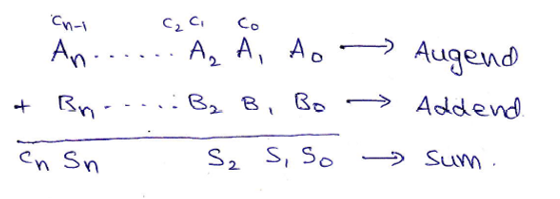Block diagram and Logic circuit diagram of a Parallel Binary adder can be given as,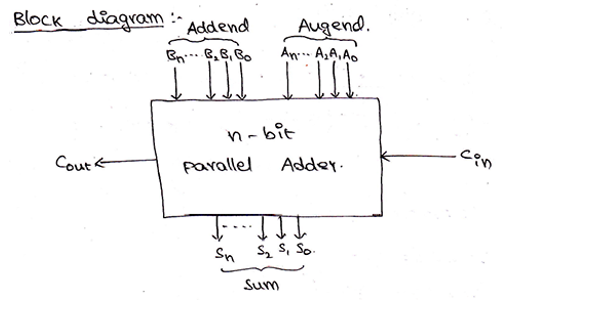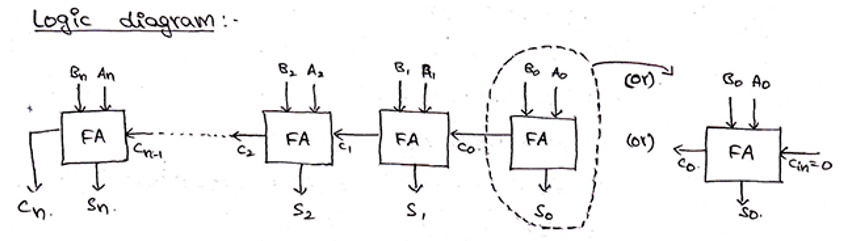From the above-provided logic, we need 4 full adders connected together to add 4-bit binary numbers. For 4-bit binary numbers A and B of the form,

A: A3 A2 A1 A0 and B: B3 B2 B1 B0, its sum can be obtained as,

A： A 3 A 2 A 1 A 0B： B 3 B 2 B 1 B 0 ，其总和可以得出，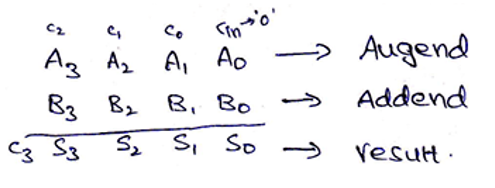Block diagram and Logic circuit diagram of a 4-bit Binary adder can be given as,

4位二进制加法器的框图和逻辑电路图可以表示为：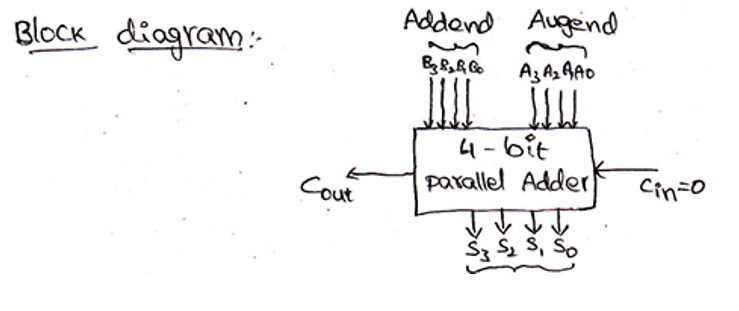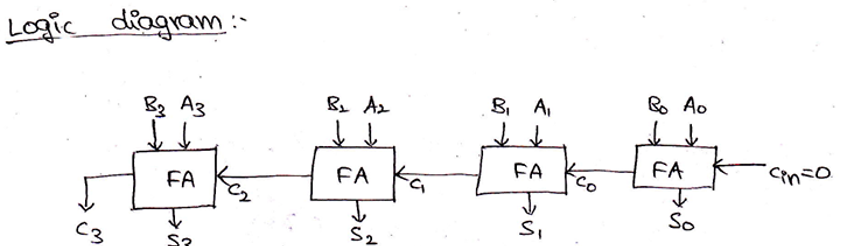### 4位二进制减法器 (4-Bit Binary Subtractor)

We already know that two numbers A (Minuend) and B (Subtrahend) can be subtracted using 2s complement method, where,

    A – B   = A + 2s complement of B
= A + 1s complement of B + 1



Thus, 4-bit binary numbers A and B can subtract as,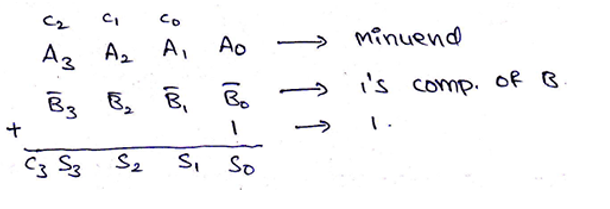And the logic circuit for the same can be drawn as,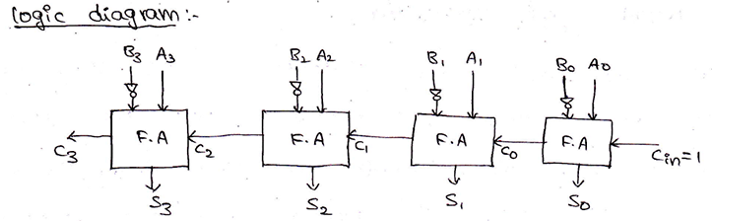Parallel Adder/Subtractor using a single circuit can be also designed using a Mod bit (M), where mod bit M decides whether the circuit will act as an adder or a subtractor. When M=0, then the circuit acts as an adder and when M=1, then the circuit acts as a subtractor. The circuit for the same can be drawn as,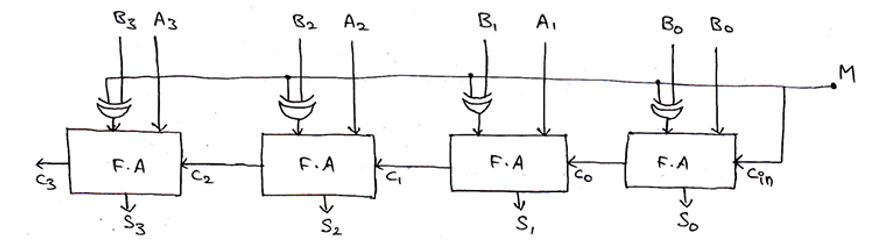When M=0,
output  = A3 A2 A1 A0 + B3 B2 B1 B0 + Cin          [Since, A⊕0 = A]
= A3 A2 A1 A0 + B3 B2 B1 B0 + 0
= A + B



Thus, circuit acts as a 4-bit binary adder.

    When M=1,
output  = A3 A2 A1 A0 + B3 B2 B1 B0 + Cin
= A3 A2 A1 A0 + B3 B2 B1 B0 + 1
= A + B + 1
= A – B



Thus, circuit acts as a 4-bit binary subtractor

05-07

07-181万+
07-01
12-015066
03-277237
03-273万+
10-072613
04-151013
10-023279
11-276262
12-231618
03-091454
03-28
04-073014
07-15757
06-049954
12-061万+
07-293634

### “相关推荐”对你有帮助么？

•非常没帮助
•没帮助
•一般
•有帮助
•非常有帮助点击重新获取扫码支付余额充值